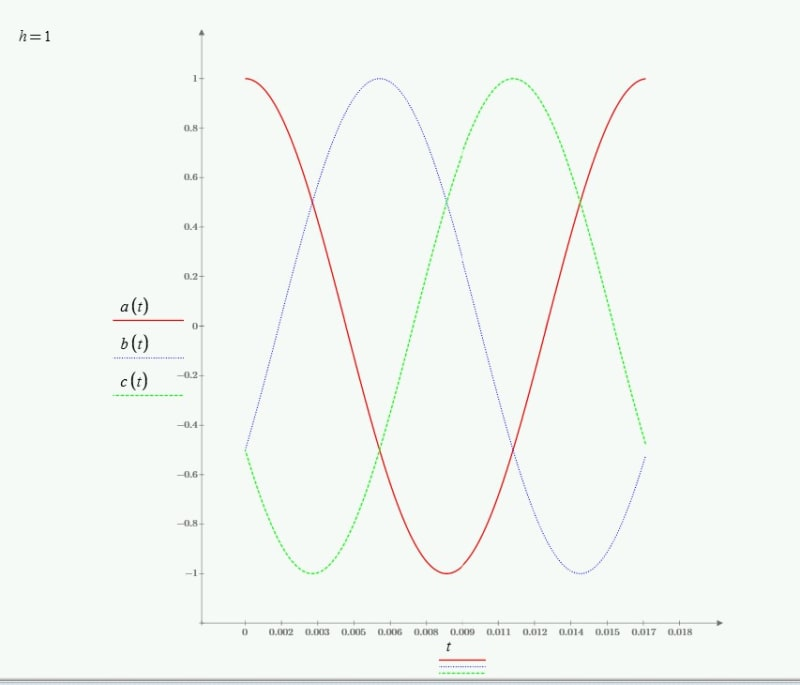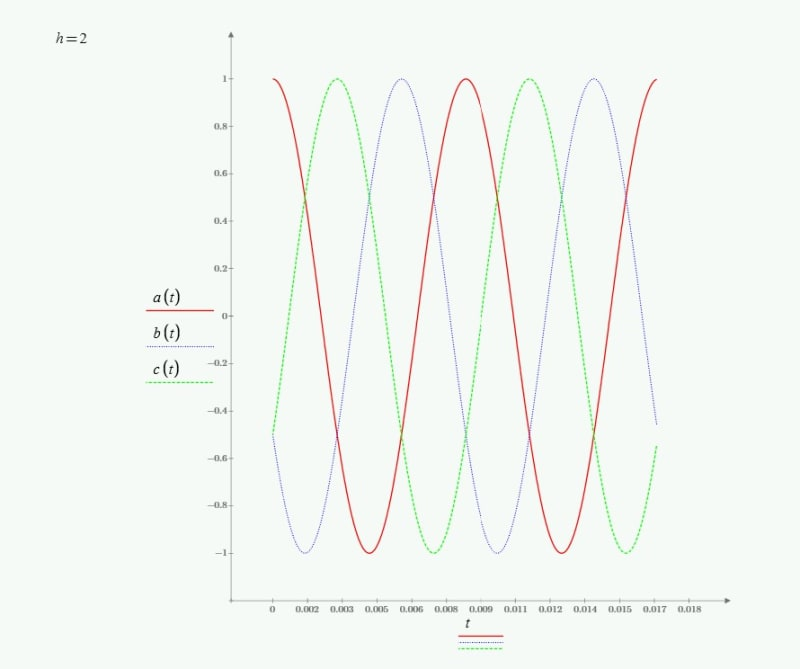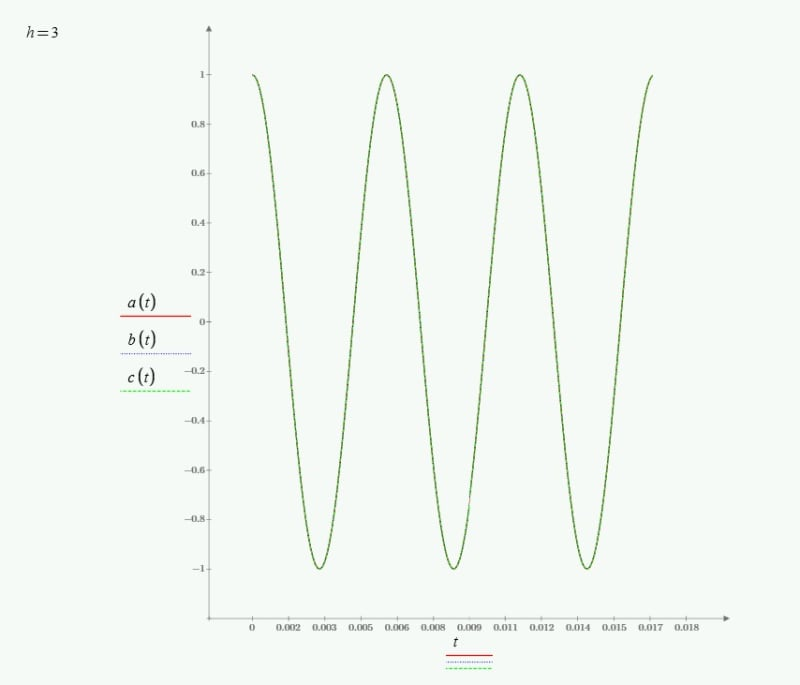×
INTELLIGENT WORK FORUMS
FOR ENGINEERING PROFESSIONALS

Are you an
Engineering professional?
Join Eng-Tips Forums!
• Talk With Other Members
• Be Notified Of Responses
• Keyword Search
Favorite Forums
• Automated Signatures
• Best Of All, It's Free!

*Eng-Tips's functionality depends on members receiving e-mail. By joining you are opting in to receive e-mail.

#### Posting Guidelines

Promoting, selling, recruiting, coursework and thesis posting is forbidden.

# Question : Rotation Direction of Ha

## Question : Rotation Direction of Ha

(OP)
Question : Rotation Direction of Harmonics. Why some CW and some CCW in 3 phase induction motor?

Assuming with A-B-C phasor sequence, a 3 phase induction motor field rotates clockwise. Assuming the motor has 4 poles, speed will be just under 1800 rpm clockwise for 60hz supply. If we decrease frequency, the motor will slow down and if we increase frequency, the motor will speed up, in the same direction. I have difficulty understanding that for 2nd harmonic, meaning at 120hz, the field rotates in opposite direction.
I am looking for some simple explanation (Not too much mathematics) to understand why 2nd harmonic (and some other harmonics) rotate in opposite direction in the motor.
I do understand the positive, Negative and zero sequence components at fundamental frequency and also it is clear that if we switch any two supply terminals of induction motor, it will rotate in opposite direction. The question is specific to 2nd harmonic (120 hz) which may have small magnitude, but why opposite direction to fundamental frequency rotation, when no terminals have been switched ?
Thanks.

### RE: Question : Rotation Direction of Ha

> I am looking for some simple explanation (Not too much mathematics) to understand why 2nd harmonic (and some other harmonics) rotate in opposite direction in the motor.

You have to assume three identical distorted (i.e. nonsinusoidal) phase waveforms shifted in time by 120 degrees of fundamental from each other. Once you make those assumptions, negative sequence second harmonic and zero sequence third harmonic are a natural consequence...

I said the distorted waveforms have a time shift among phases which equates to 120 degrees of the fundamental component. Let’s say we are in 50hz land, a cycle is 20 msec, and 120 degrees equates to 6.67msec. So we have a 6.67msec time shift among phases. That 6.67msec time shift applies to not only the fundamental but to all the harmonics. Let's look at the implications of that 6.67msec time shift for those harmonics:
• How much phase shift does that 6.67msec equate to for the 2nd harmonic? The 2nd harmonic is a period of 10msec, so 6.67msec is two thirds of a "2nd harmonic cycle", or 240 degrees. If a 120 degree phase among phases shift corresponds to forward rotation, then a 240 degree phase shift must equate to reverse rotation (assuming we have the same ordering and physical positioning of phases in both cases). If that doesn't make sense then draw three vectors labeled A1, B1, C1 separated by 120 degrees when going in the CW direction which will be assumed as forward; Now draw three vectors A2, B2, C2 separated by 240 degrees also in the CW direction.... those A2/B2/C2 vectors are in fact separated also by 120 degrees in the CCW direction which is the reverse direction... i.e. A2/B2/C2 rotate in the opposite direction as A1/B1/C1
• How much phase shift does that 6.67msec equate to for the 3rd harmonic? The period of the third harmonic is 20msec/3 = 6.67msec. How much phase shft does 6.67msec equate to for the 3rd harmonic? It is a 360 degree phase shift. That means the third harmonics on all three phases are “in phase” i.e. zero sequence.
I'm not sure if I did a good job of explaining without math but I tried. If you draw pictures of the waveforms and vectors, that would inevitably help.

=====================================
(2B)+(2B)' ?

### RE: Question : Rotation Direction of Ha

(OP)
Thanks Electricpete for very good explanation. It is interesting that motor may rotate (at least theoretically) at twice the speed, in the same direction, if frequency is doubled, i.e. from 60 hz to 120 hz. But the second harmonic ( which is also 120 hz)rotates in opposite direction. As you explained one is symmetric supply and other (harmonics) is result of distorted 3 phase supply.
...."That 6.67msec time shift applies to not only the fundamental but to all the harmonics".......I have still not fully understood the statement wrt harmonics. But I will keep trying.
I want to confirm that symmetrical components apply to only 60hz balance or unbalanced system. But harmonics is another tool to evaluate unbalanced or distorted system and symmetric components are not applied to harmonics......is that correct ? Thanks.
Best Regards.

### RE: Question : Rotation Direction of Ha

A bit of Mathcad fun I had in my files: Phases are all shifted 120 degrees based on a fundamental 60hz frequency. h is the harmonic. Note the fundamental is absent in the h=2 and 3 graphs.### RE: Question : Rotation Direction of Ha

#### Quote:

...."That 6.67msec time shift applies to not only the fundamental but to all the harmonics".......I have still not fully understood the statement wrt harmonics.

Look at phase A. It is a non-sinusoidal waveform periodic at 50hz. By Fourier theory, it can be represented as a sum of harmonics of 50hz.

Look at phase B. It is identical to phase B but it is shifted by 6.33 msec behind phase A. If the total waveform is shifted by 6.33mseconds, then each of the harmonics is also shifted by 6.33 msec.

Likewise phase C is identical but shifted another 6.33msec behind phase B. It applies to all the harmonics.

That time shift (6.33msec) equates to 1/3 of the period of the fundamental (6.33msec/20msec = 1/3 cycle of fundamental = 120 degrees of fundamental).

The same time shift (6.33msec) equates to 2/3 of the period of the second harmonic (6.33msec/10msec = 2/3 cycle of 2nd harmonic = 240 degrees of 2nd harmonic).

=====================================
(2B)+(2B)' ?

### RE: Question : Rotation Direction of Ha

(OP)
Thanks Stevenal and Electricpete. Both of you have tried to explain the idea further very nicely.
I have understood from all above, that the original 120 degree phase shift for phase B wrt A, becomes 240 degree shift for 2nd Harmonic, (and original 240 degree phase shift for phase C wrt A becomes 480 degree for 2nd Harmonic, which is 120 degree) which will reverse the rotation.
Is that correct or not ?
I am sorry that I am a slow learner, I still have some contradiction in my mind. I see the same phase shift as above, if I double the fundamental frequency, but in that case rotation will be same.
In other words, With Respect to Original time scale, can we say that phase B will lag phase A by 60 degree (instead of 120 degree) and phase C will lag phase A by 120 degree (instead of 240 degree) for 2nd harmonic ? It seems, in this case rotation does not reverse.

I know that I have understood correct answers after long thinking, and this is one of those. (So if you decide not to respond, I will understand.) Thanks again, Best Regards.

### RE: Question : Rotation Direction of Ha

#### Quote:

I have understood from all above, that the original 120 degree phase shift for phase B wrt A, becomes 240 degree shift for 2nd Harmonic, (and original 240 degree phase shift for phase C wrt A becomes 480 degree for 2nd Harmonic, which is 120 degree) which will reverse the rotation.
Is that correct or not ?

That is correct. The time shift that corresponds to 120 degrees of fundamental (6.67msec, assuming 50hz) also corresponds to 240 degrees of 2nd harmonic.

=====================================
(2B)+(2B)' ?

### RE: Question : Rotation Direction of Ha

If you double the fundamental frequency, you've simply doubled the speed of rotation. B still lags A by the same 120 degrees based on the new fundamental. This is not the same as harmonics. The h=2 graph above shows acb rotation instead of abc. Consider this a graph with the fundamental filtered out. Cosines are shifted by 120 based on fundamental.

### RE: Question : Rotation Direction of Ha

(OP)
Thanks Stevenal. Now it makes sense.
1. "The 2nd, 5th, 8th, 11th........harmonics will rotate opposite to fundamental." This statement has tobe accepted as true because this is proved mathematically for distorted waveform. As you mentioned above these harmonics follow Cosine wave ( proved mathematically........as I said earlier my Mathes knowledge is rusty so I will accept these), so that fundamental ABC sequence will become ACB for 2nd, 5th, 8th, 11th......harmonics.

Thanks Electricpete also for your effort to drive home the point.
Best Regards.

### RE: Question : Rotation Direction of Ha

I'll comment on Stevenal's figures in order to try to tie the two explanations together, because we're saying the same thing in a slightly different way.

The critical assumption for constructing his diagrams that I'd like to highlight again:

> Phases are all shifted 120 degrees based on a fundamental 60hz frequency

And equivalently all harmonics are shifted by the same time duration (call it t-delay) which corresponds to one third cycle of the fundamental = 120 deg of fundamental.

When you are attempting to convert that time duration into fraction of a cycle for each of the harmonics, you have to take into account that the period of a cycle for each harmonic (Th) is different than the period of the fundamental (T1), with the following relationships:
• T2/T1 = 1/2
• T3/T1 = 1/3
• T4/T1 = 1/4
• T5/T1 = 1/5
• T6/T1 = 1/6
So if you have a time delay between A and B equivalent to a third cycle delay on 1st harmonic, you can find out what fraction of a cycle it is for the other harmonics by taking into account those ratios:
• t-delay/T1 = 1/3 cycle of 1st harmonic = 120 degrees of first harmomnic => this is positive sequence by our definition
• t-delay/T2 = t-delay/T1 *(T1/T2) = 2/3 cycle of 2nd harmonic (240 degrees of 2nd harmonic) => negative seq
• t-delay/T3 = t-delay/T1 *(T1/T3) = 3/3 cycle of 3rd harmonic (360 degrees of 3rd harmonic) => zero seq
• t-delay/T4 = t-delay/T1 *(T1/T4) = 4/3 cycle of 4th harmonic (480-360=120 degrees of 4th harmonic) => positive seq
• t-delay/T5 = t-delay/T1 *(T1/T5) = 5/3 cycle of 5th harmonic (600-360=240 degrees of 5th harmonic) => negative seq
• t-delay/T6 = t-delay/T1 *(T1/T6) = 6/3 cycle of 6th harmonic (720-360=360 degrees of 6th harmonic) => zero seq
=====================================
(2B)+(2B)' ?

#### Red Flag This Post

Please let us know here why this post is inappropriate. Reasons such as off-topic, duplicates, flames, illegal, vulgar, or students posting their homework.

#### Red Flag Submitted

Thank you for helping keep Eng-Tips Forums free from inappropriate posts.
The Eng-Tips staff will check this out and take appropriate action.

#### Resources

Low-Volume Rapid Injection Molding With 3D Printed Molds
Learn methods and guidelines for using stereolithography (SLA) 3D printed molds in the injection molding process to lower costs and lead time. Discover how this hybrid manufacturing process enables on-demand mold fabrication to quickly produce small batches of thermoplastic parts. Download Now
Examine how the principles of DfAM upend many of the long-standing rules around manufacturability - allowing engineers and designers to place a partâ€™s function at the center of their design considerations. Download Now
Industry Perspective: Education and Metal 3D Printing
Metal 3D printing has rapidly emerged as a key technology in modern design and manufacturing, so itâ€™s critical educational institutions include it in their curricula to avoid leaving students at a disadvantage as they enter the workforce. Download Now

Close Box

# Join Eng-Tips® Today!

Join your peers on the Internet's largest technical engineering professional community.
It's easy to join and it's free.

Here's Why Members Love Eng-Tips Forums:

•Talk To Other Members
• Notification Of Responses To Questions
• Favorite Forums One Click Access
• Keyword Search Of All Posts, And More...

Register now while it's still free!